# Community

Blog Events Webinars Tutorials Forum
×
Community Blog How Mars Paves the Way for the Future of Data Science

# How Mars Paves the Way for the Future of Data Science

Here introduces Mars, Alibaba's open source distributed scientific computing engine, the commonalities and differences of code within Mars and RAPIDS.

Mars is Alibaba's first open source and independently developed computing engine for large-scale scientific computing. Developers can download and install Mars from PyPI or obtain the source code from GitHub and participate in the development.

Mars is different from existing big data computing engines, of which the computing models are mainly based on relational algebra. Mars introduces distributed technologies into the scientific computing/numerical computation field and significantly improves the computing scale and efficiency of scientific computing. Currently Mars has been applied to both business and production scenarios at Alibaba or for its customers on the cloud.

## Mars – Alibaba's Open Source Distributed Scientific Computing Engine

### Overview

Scientific computing, or numerical computation, is a rapidly growing field that uses computers to solve numerical computation problems in scientific research and engineering. Scientific computing is applied in a variety of fields such as image processing, machine learning, and deep learning. Many scientific computing tools are available for different languages and libraries. Among these tools, NumPy stands out with its simple and easy-to-use syntax and powerful performance and contributes to a large technology stack that is based on NumPy itself ( as shown in the following picture).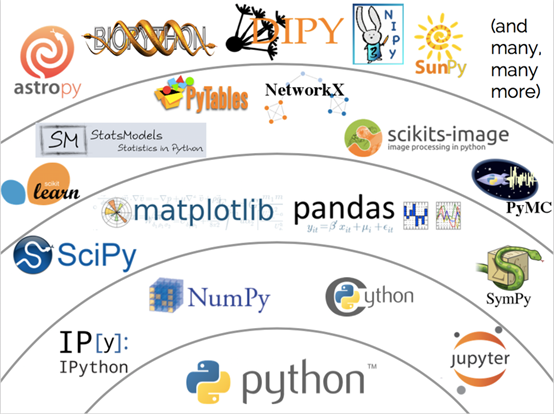Multidimensional arrays are a core concept of NumPy, and are the foundation for various upper-layer tools. A multidimensional array is also called a tensor. Compared with two-dimensional tables or matrices, tensors have more powerful expressiveness. Therefore, popular deep learning frameworks usually adopt data structures that are based on tensors.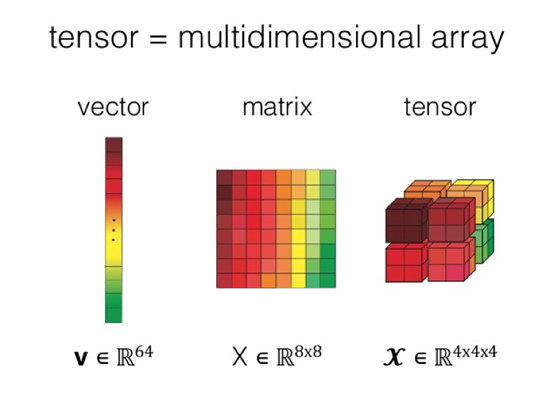With increasing trends in machine learning and deep learning, the tensor concept is becoming more ubiquitous and the need for general-purpose computing on tensors also increases. However, the reality is that, as powerful as NumPy is, this scientific computing library is still used on a single machine and cannot break the performance bottlenecks. Currently popular distributed computing engines are not designed specifically for scientific computing. The inconsistent upper-layer interfaces make it hard to write scientific computing tasks in traditional SQL/MapReduce. These engines are not optimized for scientific computing and therefore provide unsatisfactory computing efficiency.

Understanding the aforementioned problems currently encountered in the scientific computing field, the Alibaba Cloud MaxCompute R&D team finally broke the boundary between big data and scientific computing, built the first version of Mars and published its open source code after over one year of research and development. Mars is a universal distributed computing framework based on tensors. Mars makes it possible to perform large-scale scientific computing tasks using only several lines of code, whereas using MapReduce requires hundreds of lines of code.

In addition, Mars can significantly improve computing performance. Currently Mars has implemented tensors, that is, Mars has basically implemented the distribution of NumPy with 70% of the common NumPy interfaces already available. Mars 0.2 is implementing the distribution of Pandas and will soon provide fully compatible Pandas interfaces to build the whole ecology.

As a new-generation ultra-large scientific computing engine, Mars not only accelerates scientific computing into the "distributed" era but also makes it possible to perform efficient scientific computing on big data.

### Core Features of Mars

Compatibility with Familiar Interfaces

Mars provides interfaces that are compatible with NumPy by using the tensor module. Users can transplant their code logic into Mars simply by replacing and importing their existing code written in NumPy. By doing so, users can implement a scale that is tens of thousands of times larger than the original scale as well as increase processing capacity by several tens of times. Mars has implemented around 70% of the common NumPy interfaces.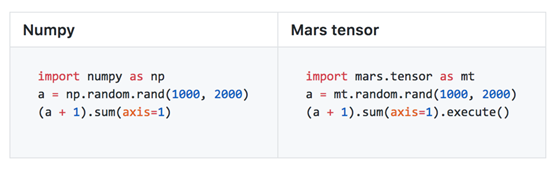Full Exploitation of GPU Acceleration

Additionally, Mars also expands NumPy and makes full advantage of existing GPU achievements in the scientific field. When creating a tensor, let subsequent computing tasks run on GPUs simply by specifying gpu=True. Example:

``````a = mt.random.rand(1000, 2000, gpu=True)# Specifies creation on GPU
(a + 1).sum(axis=1).execute()
``````

Sparse Matrix

Mars also supports two-dimensional sparse matrices. To create a sparse matrix, simply specify sparse=True. Take the eye interface for example. This interface creates a unit diagonal matrix in which entries outside the main diagonal are all 0 and the diagonal entries are all 1. So sparse matrix storage can be used.

``````a = mt.eye(1000, sparse=True) # Creates a sparse matrix
(a + 1).sum(axis=1).execute()
``````

System Design

This section describes the system design of Mars to show you how Mars enables parallel and automated scientific computing tasks and powerful performance.

Splitting - Tile

Mars typically splits scientific computing tasks. If a tensor is given, Mars will automatically split it by each dimension into small chunks and then process these chunks separately. Automatic splitting and task parallelism are supported for all operators implemented in Mars. This automatic splitting is called Tiling in Mars.

For example, consider a 1000×2000 tensor. If each chunk for each dimension is 500×500, then this tensor will be tiled into 8 (2×4) chunks. The tile operation will also be automatically performed for subsequent operators such as Add and SUM. The operation tiling for this example tensor is shown in the following diagram.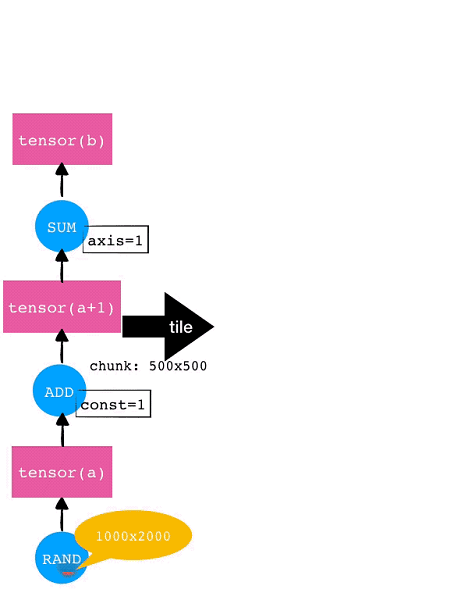Delayed Execution and Fusion Optimization

Currently it is required to explicitly invoke "execute" in order to trigger code written in Mars. This is called the Mars-based delayed execution mechanism. Users don't need to perform any actual data computing tasks when writing intermediate code. This allows users to make more optimizations for the intermediate process, enabling more optimized task execution. Currently the main optimization method in Mars is the fusion optimization, that is, to merge multiple operations into one operation and then execute that operation.

In the preceding example, after the tile operation is completed, Mars performs the fusion optimization targeting fine-grained chunk-level graphs. For example, each of the eight chunks respectively for RAND, ADD, and SUM can form one node separately. On one hand, this allows generating acceleration code by invoking libraries like NumExpr; on the other hand, reducing the number of running nodes can significantly reduce the overhead of the scheduling execution graphs.

Multiple Scheduling Methods

Mars supports a variety of scheduling methods:

1. Multi-thread mode: Mars can use multiple threads to locally schedule and execute chunk-level graphs. In NumPy, most operators are executed by using a single thread. This scheduling method alone can allow Mars to implement tiled execution graphs on a single machine and break the memory limit of a single machine in NumPy. This method can ensure that all CPU/GPU resources can be fully utilized and enable much faster performance than NumPy.
2. Single-machine/cluster mode: Mars can start the whole distributed runtime on a single machine and use multiple processes to accelerate task execution. This mode is suitable for development and debugging targeting distributed environments.
3. Distributed: Mars can start one or more schedulers and multiple workers. A scheduler will schedule chunk-level operators so that they are executed in individual workers.

The distributed execution architecture of Mars is shown in the following diagram: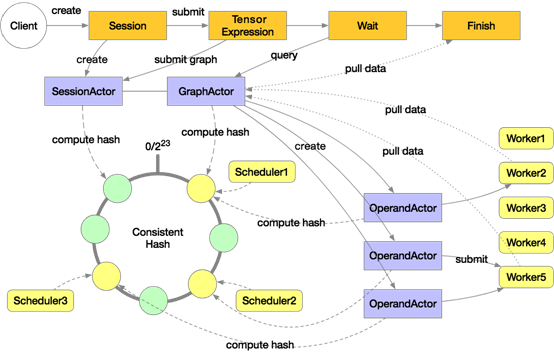A distributed execution in Mars will start multiple schedulers and workers. The preceding diagram includes three schedulers and five workers. These schedulers make up a consistent hash loop. When a user explicitly or implicitly creates a session on the client, a SessionActor is assigned to a scheduler according to the consistent hash. Then when the user submits a tensor computing task by using "execute", a GraphActor is created to manage the execution of this tensor. This tensor will be tiled into chunk-level graphs in the GraphActor.

Take three chunks for example. Three OperandActors will be created on the scheduler respectively for the three chunks. These OperandActors will be submitted to individual workers for execution, depending on whether the dependencies of these OperandActors are completed and whether cluster resources are sufficient. After this process is completed on all the OperandActors, the GraphActor will be informed that the task has been completed, then the client can pull data to display or draw graphs.

Scaling In and Out

The flexible execution graphs and multiple scheduling modes in Mars can allow code in Mars to flexibly scale in and scale out. Scale in to a single machine and use multiple cores to perform scientific computing tasks; scale out to distributed clusters to allow hundreds of workers to complete tasks that otherwise could never be done on a single machine.

## Use Mars with RAPIDS to Accelerate Data Science on GPUs in Parallel Mode

This blog explains the commonalities and differences of code within common Python tools, Mars, and RAPIDS, and how it can pave the way for the future of data science.

### Mars: Parallel and Distributed Accelerator for NumPy, Pandas, and Scikit-learn

The data science stack of Python is powerful but it has the following problems:

1. Multi-core capabilities can be used for new operations in these libraries.
2. As deep learning becomes more popular, more and more new hardware for accelerating data science is emerging. The most common hardware is the graphics processing unit (GPU) but can we use GPUs to accelerate data processing in the preorder process of deep learning?
3. The operations of these libraries are all imperative, not declarative. Focusing on telling the system how to do something, imperative operations can obtain results immediately, facilitating result exploring. Imperative operations are flexible but occupy a lot of memory resources that cannot be released quickly. Imperative operations are separated from each other. Operator fusion cannot be performed to improve performance. In contrast, declarative operations focus on telling the system what to do and are more concerned with the results. Typical declarative operations such as SQL statements and TensorFlow 1.x operations can be performed only when the results are truly needed. This is a lazy evaluation. During the process, a lot of optimization can be performed to improve performance. However, declarative operations are not flexible and are hard to debug.

To solve these problems, Mars was developed by the MaxCompute team. It aims to enable data science libraries such as NumPy, pandas, and scikit-learn to be executed in a parallel and distributed manner, so that the multi-core capabilities and new hardware can be fully utilized.

During the development of Mars, we focused on the following features:

1. We wanted Mars to be simple enough so that anyone who knows NumPy, pandas, or scikit-learn can use Mars.
2. We wanted the functions or features of these libraries to be reusable after the libraries are scheduled to multiple cores or multiple workers.
3. We wanted to allow users to switch between declarative operations and imperative operations to achieve both flexibility and performance.
4. Mars should be robust enough for production and able to cope with various failover.

These were our goals and direction of our efforts.

### Mars Tensor: Parallel and Distributed Accelerator for NumPy

As mentioned above, we wanted to make it easy for anyone who knows NumPy, pandas, or scikit-learn to use Mars. Let's use Monte Carlo as an example:

``````import mars.tensor as mt

N = 10 ** 10

data = mt.random.uniform(-1, 1, size=(N, 2))
inside = (mt.sqrt((data ** 2).sum(axis=1)) < 1).sum()
pi = (4 * inside / N).execute()
print('pi: %.5f' % pi)
``````

As you can see, `import numpy as np` is changed to `import mars.tensor as mt`. All subsequent instances of `np.`
are changed to `mt.` and the `.execute()` method is called before pi is printed.

That means, by default, Mars migrates code in a declarative manner with very low costs. You can use the `.execute()` method to get data when needed. This can maximize performance and reduce memory consumption.

Here, we have also expanded the data scale by a factor of 1,000 to 10 billion points. It took 757 milliseconds to process 10 million points (1/1000) on my notebook. Now, the data volume increases by a factor of 1,000 and 150 GB memory is required for processing `data`. The whole task cannot be completed by NumPy alone. In contrast, Mars spends only 3 minutes and 44 seconds on computing, with a peak memory usage of only 1 GB. Assuming that the memory size is infinitely large, the time required by NumPy is more than 12 minutes, an increase by a factor of 1,000. In contrast, Mars can make full use of multi-core capabilities and use declarative operations to greatly reduce the memory usage.

As mentioned above, we have tried to use both the declarative and imperative styles. To use the imperative style, we only need to configure an option at the beginning of the code.

``````import mars.tensor as mt
from mars.config import options

options.eager_mode = True  # 打开 eager mode 后，每一次调用都会立即执行，行为和 Numpy 就完全一致

N = 10 ** 7

data = mt.random.uniform(-1, 1, size=(N, 2))
inside = (mt.linalg.norm(data, axis=1) < 1).sum()
pi = 4 * inside / N  # 不需要调用 .execute() 了
print('pi: %.5f' % pi.fetch())  # 目前需要 fetch() 来转成 float 类型，后续我们会加入自动转换
``````

### Mars DataFrame: Parallel and Distributed Accelerator for pandas

Migrating code from pandas to Mars DataFrame is similar to migrating code from NumPy to Mars tensor, with only two differences. Let's use the MovieLens code as an example:

``````import mars.dataframe as md

ratings.groupby('userId').agg({'rating': ['sum', 'mean', 'max', 'min']}).execute()
``````

### Mars Learn: Parallel and Distributed Accelerator for Scikit-learn

Migrating code from scikit-learn to Mars Learn is also similar. Mars Learn only supports a few scikit-learn algorithms but we are working hard to migrate the algorithm code to it. Anyone interested is welcome to join us.

``````import mars.dataframe as md
from mars.learn.neighbors import NearestNeighbors

df = md.read_csv('data.csv')  # 输入是 CSV 文件，包含 20万个向量，每个向量10个元素
nn = NearestNeighbors(n_neighbors=10)
nn.fit(df)  # 这里 fit 的时候也会整体触发执行，因此机器学习的高层接口都是立即执行的
neighbors = nn.kneighbors(df).fetch()  # kneighbors 也已经触发执行，只需要 fetch 数据
``````

Note that Mars Learn can immediately run the fit and predict APIs for machine learning to ensure the semantic correctness.

### RAPIDS: Data Science on GPUs

You may have noticed that, so far, we have not mentioned GPUs. Now, let's talk about RAPIDS, a GPU-accelerated data science platform.

Although Compute Unified Device Architecture (CUDA) has greatly reduced the difficulty of GPU programming, it is almost impossible for data scientists to use a GPU to process the data that NumPy and pandas can process. Fortunately, NVIDIA provides an open-source RAPIDS platform. Similar to Mars, RAPIDS allows you to migrate code from NumPy, pandas, and scikit-learn to a GPU through import statements.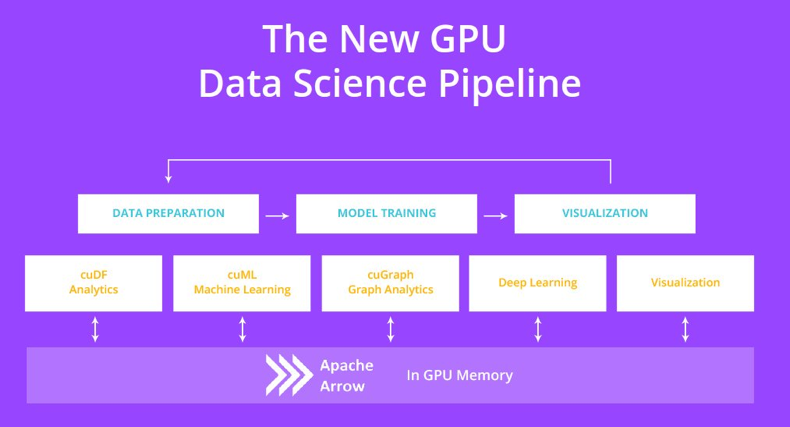RAPIDS cuDF is used to accelerate pandas, while RAPIDS cuML is used to accelerate scikit-learn.

For NumPy, CuPy can be accelerated by using GPUs, allowing RAPIDS to focus on other parts of data science.

CuPy: GPU-based Accelerator of NumPy

Let's use Monte Carlo as an example to calculate pi:

``````import cupy as cp

N = 10 ** 7

data = cp.random.uniform(-1, 1, size=(N, 2))
inside = (cp.sqrt((data ** 2).sum(axis=1)) < 1).sum()
pi = 4 * inside / N
print('pi: %.5f' % pi)
``````

In my test, CuPy reduced the CPU time consumption by more than 2000%. It dropped from 757 milliseconds to 36 milliseconds since a GPU is suitable for computing-intensive tasks.

RAPIDS cuDF: GPU-based Accelerator of Pandas

The code `import pandas as pd`
is changed to `import cudf`. You do not need to be concerned with the parallel implementation in the GPU or CUDA programming.

``````import cudf

ratings.groupby('userId').agg({'rating': ['sum', 'mean', 'max', 'min']})
``````

The runtime is reduced by over 1000%, from 18s on the CPU to 1.66s on a GPU.

RAPIDS cuML: GPU-based Accelerator of Scikit-learn

Let's continue using KNN as an example:

``````import cudf
from cuml.neighbors import NearestNeighbors

nn = NearestNeighbors(n_neighbors=10)
nn.fit(df)
neighbors = nn.kneighbors(df)
``````

The runtime is reduced from 1 minute and 52 seconds on a CPU to 17.8 seconds on a GPU.

### Benefits of Using Mars with RAPIDS

RAPIDS implements Python data science on a GPU, greatly improving the runtime efficiency for data science operations. They also use the imperative style. When Mars and RAPIDS are used together, less memory is consumed in the process, allowing more data to be processed. Mars can also distribute computing to multiple workers and GPUs to improve the data scale and computing efficiency.

It is easy to use a GPU in Mars. You only need to specify gpu=True for corresponding functions. For example, a GPU can be used to create tensors and read CSV files.

``````import mars.tensor as mt
import mars.dataframe as md

a = mt.random.uniform(-1, 1, size=(1000, 1000), gpu=True)
``````

The following figure shows how Mars accelerates pi calculation in scale-up and scale-out dimensions by using Monte Carlo methods. Generally, we can accelerate a data science task in either of two ways: Scale-up means using better hardware, such as a better CPU, a larger memory, or a GPU, while scale-out means using more workers to improve efficiency in a distributed manner.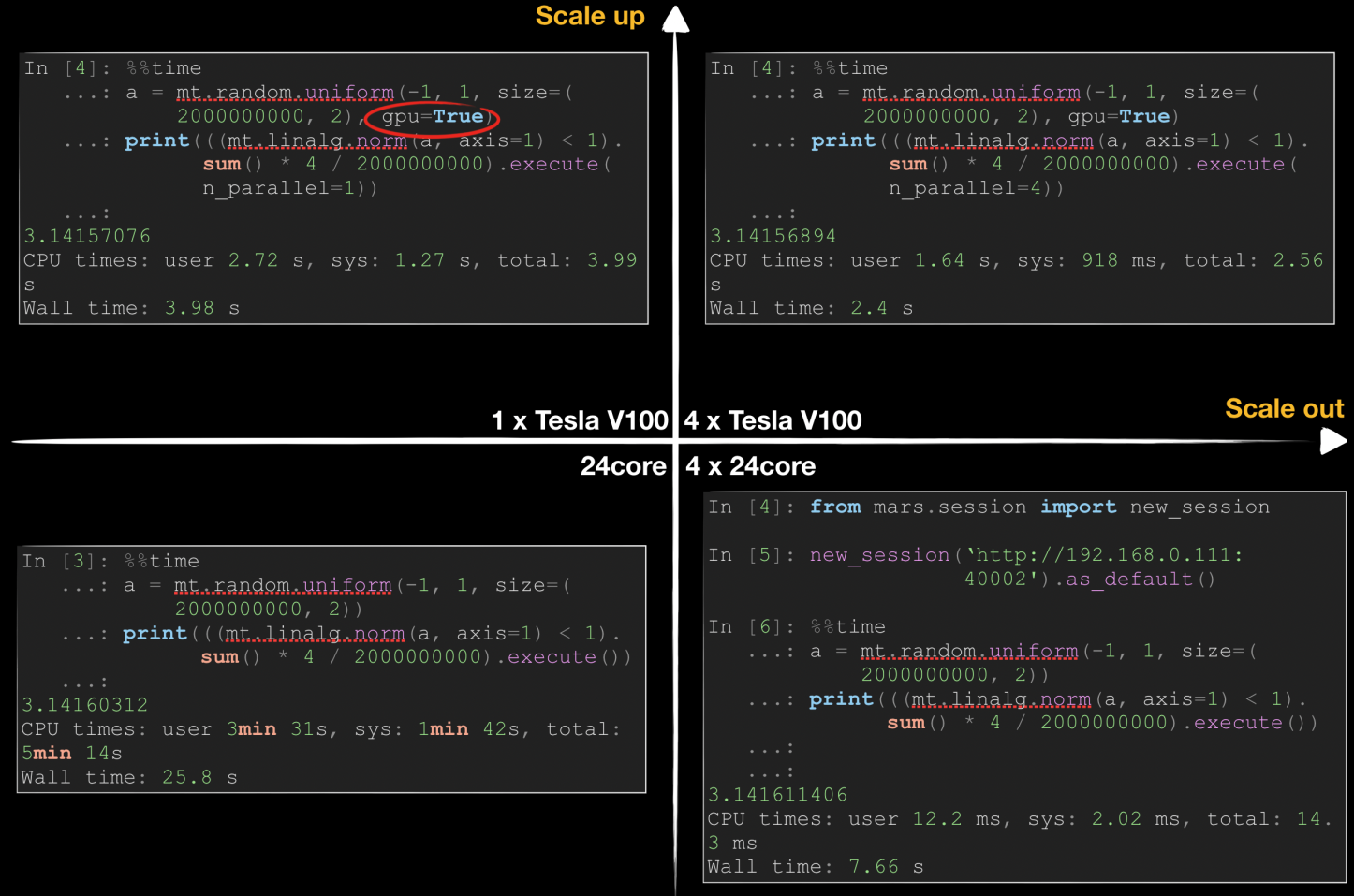As shown in the preceding figure, Mars requires 25.8 seconds for computing on one 24-core server, while the time is linearly reduced in distributed mode when four 24-core servers are used. By using NVIDIA Tesla V100, we can reduce the runtime on a single server to 3.98 seconds, which surpasses the performance of 4 CPUs. By using multiple GPUs, we can further reduce the runtime. It is difficult to linearly reduce the runtime because the network and data replication overhead increase significantly.

## Related Product

### MaxCompute

MaxCompute (previously known as ODPS) is a general purpose, fully managed, multi-tenancy data processing platform for large-scale data warehousing. MaxCompute supports various data importing solutions and distributed computing models, enabling users to effectively query massive datasets, reduce production costs, and ensure data security.

## Related Documentation

### Build an online operation analysis platform

This tutorial describes an end-to-end solution for you to use big data services of Alibaba Cloud to build an online operational analytics platform based on the basic requirements for such a platform in the big data era. This solution allows you to write data to a storage system at high concurrency, process data efficiently, and analyze and display data.

0 0 0
Share on